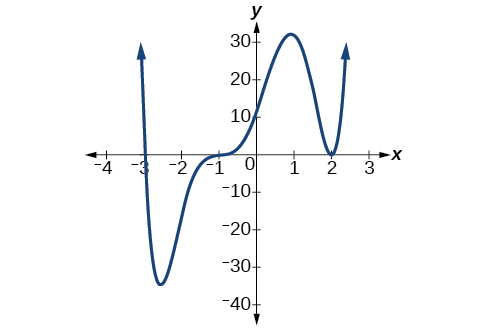# 5.3 Graphs of polynomial functions  (Page 2/13)

 Page 2 / 13

## Finding the x -intercepts of a polynomial function by factoring

Find the x -intercepts of $\text{\hspace{0.17em}}f\left(x\right)={x}^{6}-3{x}^{4}+2{x}^{2}.$

We can attempt to factor this polynomial to find solutions for $\text{\hspace{0.17em}}f\left(x\right)=0.$

$\begin{array}{ccccccccccc}& & & & \hfill \left({x}^{2}-1\right)& =& 0\hfill & & \hfill \left({x}^{2}-2\right)& =& 0\hfill \\ \hfill {x}^{2}& =& 0\hfill & \phantom{\rule{2em}{0ex}}\text{or}& \hfill {x}^{2}& =& 1\hfill & \phantom{\rule{2em}{0ex}}\text{or}& \hfill {x}^{2}& =& 2\hfill \\ \hfill x& =& 0\hfill & & \hfill x& =& ±1\hfill & & \hfill x& =& ±\sqrt{2}\hfill \end{array}$

This gives us five x -intercepts: $\text{\hspace{0.17em}}\left(0,0\right),\left(1,0\right),\left(-1,0\right),\left(\sqrt{2},0\right),\text{\hspace{0.17em}}$ and $\text{\hspace{0.17em}}\left(-\sqrt{2},0\right).\text{\hspace{0.17em}}$ See [link] . We can see that this is an even function because it is symmetric about the y -axis.

## Finding the x -intercepts of a polynomial function by factoring

Find the x -intercepts of $\text{\hspace{0.17em}}f\left(x\right)={x}^{3}-5{x}^{2}-x+5.$

Find solutions for $\text{\hspace{0.17em}}f\left(x\right)=0\text{\hspace{0.17em}}$ by factoring.

$\begin{array}{ccccccccccc}\hfill x+1& =& 0\hfill & \phantom{\rule{2em}{0ex}}\text{or}\phantom{\rule{2em}{0ex}}& \hfill x-1& =& 0\hfill & \phantom{\rule{2em}{0ex}}\text{or}\phantom{\rule{2em}{0ex}}& \hfill x-5& =& 0\hfill \\ \hfill x& =& -1\hfill & & \hfill x& =& 1\hfill & & \hfill x& =& 5\hfill \end{array}$

There are three x -intercepts: $\text{\hspace{0.17em}}\left(-1,0\right),\left(1,0\right),\text{\hspace{0.17em}}$ and $\text{\hspace{0.17em}}\left(5,0\right).\text{\hspace{0.17em}}$ See [link] .

## Finding the y - and x -intercepts of a polynomial in factored form

Find the y - and x -intercepts of $\text{\hspace{0.17em}}g\left(x\right)={\left(x-2\right)}^{2}\left(2x+3\right).$

The y -intercept can be found by evaluating $\text{\hspace{0.17em}}g\left(0\right).$

$\begin{array}{ccc}\hfill g\left(0\right)& =& {\left(0-2\right)}^{2}\left(2\left(0\right)+3\right)\hfill \\ & =& 12\hfill \end{array}$

So the y -intercept is $\text{\hspace{0.17em}}\left(0,12\right).$

The x -intercepts can be found by solving $\text{\hspace{0.17em}}g\left(x\right)=0.$

${\left(x-2\right)}^{2}\left(2x+3\right)=0$
$\begin{array}{ccccccc}\hfill {\left(x-2\right)}^{2}& =& 0\hfill & & \hfill \left(2x+3\right)& =& 0\hfill \\ \hfill x-2& =& 0\hfill & \phantom{\rule{2em}{0ex}}\text{or}\phantom{\rule{2em}{0ex}}& \hfill x& =& -\frac{3}{2}\hfill \\ \hfill x& =& 2\hfill & & & & \end{array}$

So the x -intercepts are $\text{\hspace{0.17em}}\left(2,0\right)\text{\hspace{0.17em}}$ and $\text{\hspace{0.17em}}\left(-\frac{3}{2},0\right).$

## Finding the x -intercepts of a polynomial function using a graph

Find the x -intercepts of $\text{\hspace{0.17em}}h\left(x\right)={x}^{3}+4{x}^{2}+x-6.$

This polynomial is not in factored form, has no common factors, and does not appear to be factorable using techniques previously discussed. Fortunately, we can use technology to find the intercepts. Keep in mind that some values make graphing difficult by hand. In these cases, we can take advantage of graphing utilities.

Looking at the graph of this function, as shown in [link] , it appears that there are x -intercepts at $\text{\hspace{0.17em}}x=-3,-2,\text{\hspace{0.17em}}$ and $\text{\hspace{0.17em}}1.$

We can check whether these are correct by substituting these values for $\text{\hspace{0.17em}}x\text{\hspace{0.17em}}$ and verifying that

$h\left(-3\right)=h\left(-2\right)=h\left(1\right)=0$

Since $\text{\hspace{0.17em}}h\left(x\right)={x}^{3}+4{x}^{2}+x-6,\text{\hspace{0.17em}}$ we have:

$\begin{array}{ccc}\hfill h\left(-3\right)& =& {\left(-3\right)}^{3}+4{\left(-3\right)}^{2}+\left(-3\right)-6=-27+36-3-6=0\hfill \\ \hfill h\left(-2\right)& =& {\left(-2\right)}^{3}+4{\left(-2\right)}^{2}+\left(-2\right)-6=-8+16-2-6=0\hfill \\ \hfill h\left(1\right)& =& {\left(1\right)}^{3}+4{\left(1\right)}^{2}+\left(1\right)-6=1+4+1-6=0\hfill \end{array}$

Each x -intercept corresponds to a zero of the polynomial function and each zero yields a factor, so we can now write the polynomial in factored form.

$\begin{array}{ccc}\hfill h\left(x\right)& =& {x}^{3}+4{x}^{2}+x-6\hfill \\ & =& \left(x+3\right)\left(x+2\right)\left(x-1\right)\hfill \end{array}$

Find the y - and x -intercepts of the function $\text{\hspace{0.17em}}f\left(x\right)={x}^{4}-19{x}^{2}+30x.$

y -intercept $\text{\hspace{0.17em}}\left(0,0\right);\text{\hspace{0.17em}}$ x -intercepts $\text{\hspace{0.17em}}\left(0,0\right),\left(–5,0\right),\left(2,0\right),\text{\hspace{0.17em}}$ and $\text{\hspace{0.17em}}\left(3,0\right)$

## Identifying zeros and their multiplicities

Graphs behave differently at various x -intercepts. Sometimes, the graph will cross over the horizontal axis at an intercept. Other times, the graph will touch the horizontal axis and "bounce" off.

Suppose, for example, we graph the function shown.

$f\left(x\right)=\left(x+3\right){\left(x-2\right)}^{2}{\left(x+1\right)}^{3}$

Notice in [link] that the behavior of the function at each of the x -intercepts is different.Identifying the behavior of the graph at an x -intercept by examining the multiplicity of the zero.

The x -intercept $\text{\hspace{0.17em}}x=-3\text{\hspace{0.17em}}$ is the solution of equation $\text{\hspace{0.17em}}\left(x+3\right)=0.\text{\hspace{0.17em}}$ The graph passes directly through the x -intercept at $\text{\hspace{0.17em}}x=-3.\text{\hspace{0.17em}}$ The factor is linear (has a degree of 1), so the behavior near the intercept is like that of a line—it passes directly through the intercept. We call this a single zero because the zero corresponds to a single factor of the function.

#### Questions & Answers

x exposant 4 + 4 x exposant 3 + 8 exposant 2 + 4 x + 1 = 0
x exposent4+4x exposent3+8x exposent2+4x+1=0
HERVE
How can I solve for a domain and a codomains in a given function?
ranges
EDWIN
Thank you I mean range sir.
Oliver
proof for set theory
don't you know?
Inkoom
find to nearest one decimal place of centimeter the length of an arc of circle of radius length 12.5cm and subtending of centeral angle 1.6rad
factoring polynomial
find general solution of the Tanx=-1/root3,secx=2/root3
find general solution of the following equation
Nani
the value of 2 sin square 60 Cos 60
0.75
Lynne
0.75
Inkoom
when can I use sin, cos tan in a giving question
depending on the question
Nicholas
I am a carpenter and I have to cut and assemble a conventional roof line for a new home. The dimensions are: width 30'6" length 40'6". I want a 6 and 12 pitch. The roof is a full hip construction. Give me the L,W and height of rafters for the hip, hip jacks also the length of common jacks.
John
I want to learn the calculations
where can I get indices
I need matrices
Nasasira
hi
Raihany
Hi
Solomon
need help
Raihany
maybe provide us videos
Nasasira
Raihany
Hello
Cromwell
a
Amie
What do you mean by a
Cromwell
nothing. I accidentally press it
Amie
you guys know any app with matrices?
Khay
Ok
Cromwell
Solve the x? x=18+(24-3)=72
x-39=72 x=111
Suraj
Solve the formula for the indicated variable P=b+4a+2c, for b
Need help with this question please
b=-4ac-2c+P
Denisse
b=p-4a-2c
Suddhen
b= p - 4a - 2c
Snr
p=2(2a+C)+b
Suraj
b=p-2(2a+c)
Tapiwa
P=4a+b+2C
COLEMAN
b=P-4a-2c
COLEMAN
like Deadra, show me the step by step order of operation to alive for b
John
A laser rangefinder is locked on a comet approaching Earth. The distance g(x), in kilometers, of the comet after x days, for x in the interval 0 to 30 days, is given by g(x)=250,000csc(π30x). Graph g(x) on the interval [0, 35]. Evaluate g(5)  and interpret the information. What is the minimum distance between the comet and Earth? When does this occur? To which constant in the equation does this correspond? Find and discuss the meaning of any vertical asymptotes.
The sequence is {1,-1,1-1.....} hasByByByByBy David Martin